Enable Dark Mode!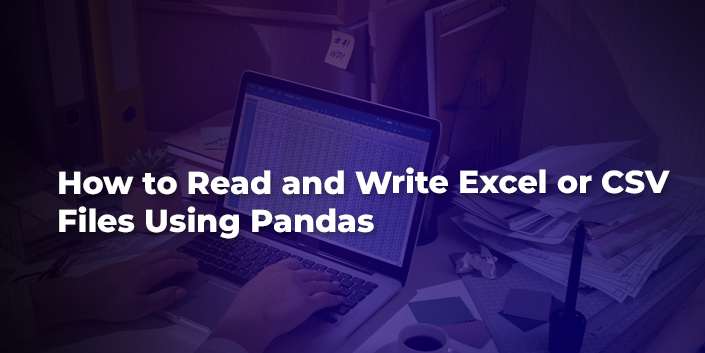By: Sahla Sherin

# How to Read and Write Excel or CSV Files Using Pandas

## Technical

Pandas is a powerful and popular library that provides high-performance data structures, data analysis tools, and manipulation tools. It was developed by Wes McKinney in 2008.

The name PANDAS is derived from “Panel Data” and “Python Data Analysis”

Used to analyze big data, get a conclusion from that data, and clean the messy data. Pandas take the value from CSV, TSV, or  SQL and will generate Python objects in rows and columns. Pandas is a Python library that makes data science very simple.

To install the pandas in Windows using the command prompt, enter the following

`# Run the following command in your pip install pandaspip install pandas(or)pip3 install pandas`

After that, you have to import the pandas. Here I’m importing the pandas as pd. ow pd contains the Pandas module

`import pandas as pd`

Then, you have to add the file that needs to be read.

`df = pd.read_excel(r'/home/cybrosys/Downloads/Financial Sample.xlsx')`

It returns the data frame object, which is a core part of the pandas. Now let’s check the data frame object by printing the df.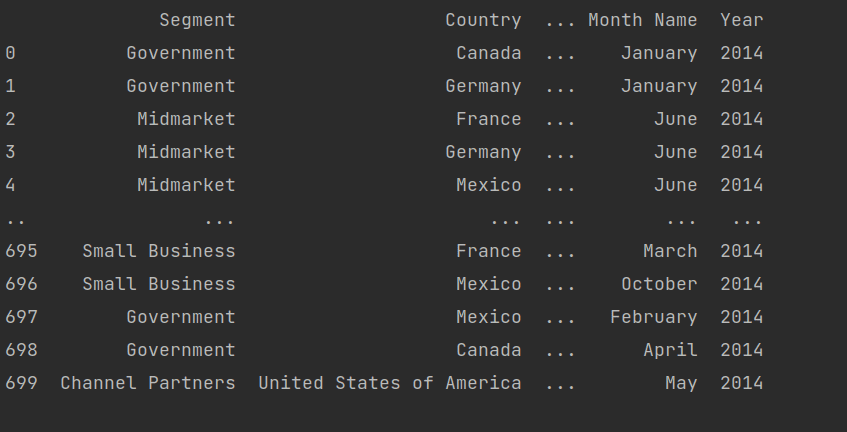We get the table values.

We can fetch the values according to our needs. Currently, I’m taking a financial sample Excel sheet.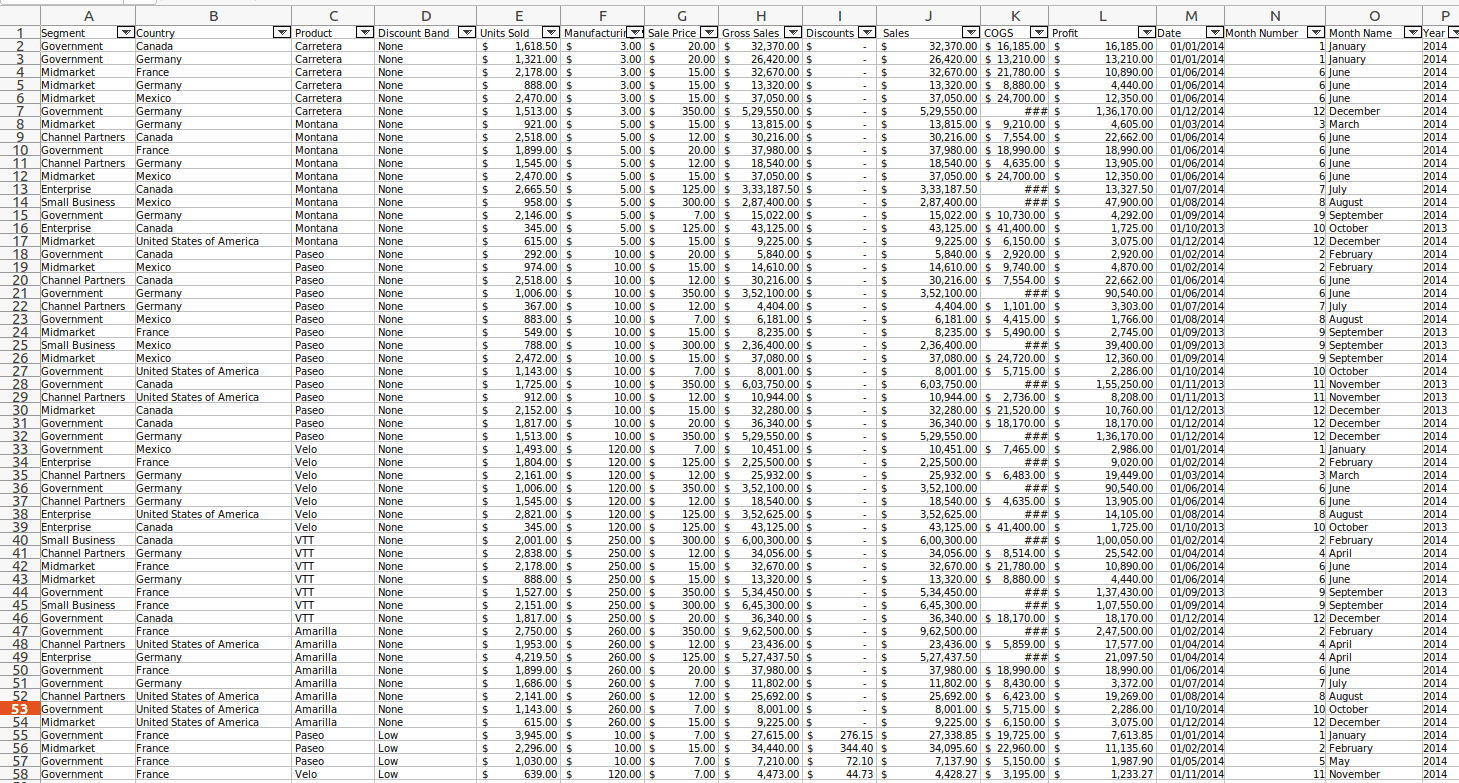Let’s check the maximum sale price from this Excel sheet, so we have to call the max to get that. The sale price is the column name.

`print(df['Sale Price'].max())`

And the result is 350

Let’s search for the segments that have a sale price is = 350

`print(df['Segment'][df['Sale Price']== 8])`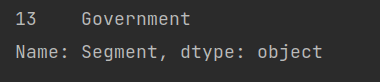The results obtained by pandas are shown above.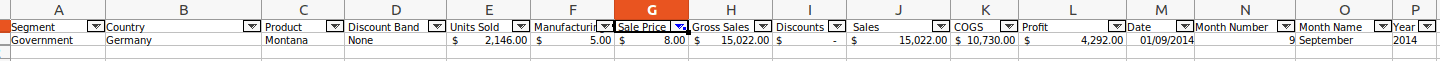This is from an Excel sheet manually.

In pandas, there exist numerous methods like max, mean, compare, count, etc

Using Pandas, we can also clean up the messy data; this process is known as data munging or data wrangling. This is very useful for data scrapping or data analytics.

For example,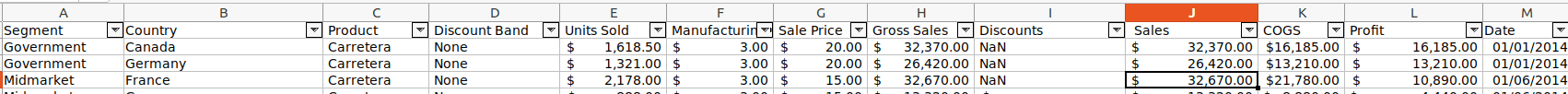In the Excel sheet, the value is NaN now filled with Zero values.

Using Pandas, we can convert the dictionary to DataFrame, for example,

`weather_data = {   'day': ['1/1/2017', '1/2/2017', '1/3/2017', '1/4/2017', '1/5/2017', '1/6/2017'],   'temperature': [32, 35, 28, 24, 32, 31],   'windspeed': [6, 7, 2, 7, 4, 2],   'event': ['Rainy', 'Sunny', 'Snow', 'Snow', 'Rainy', 'Sunny']}`

This weather_data is the dictionary. Now let’s check how to convert them to DataFrame. For that, we can use this code.

`df = pd.DataFrame(weather_data)`

Let’s print the df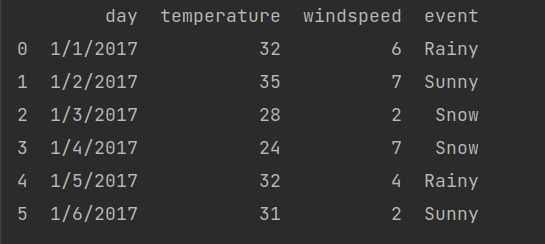We can find the number of rows and columns by using this code; For that, we just need to write .shape

Eg:-

`print(df.shape)`

The result is (6, 4)

When we are executing the function head(), then we will get the initial few rows. In addition, it is helpful in the case of big Excel sheets.

For instance, If there is an Excel sheet with 699 records and when rendering the function head(); it will generate the sample of the Excel with 4 records and also mention the number of the needed records like df.head(2) then 2 records are printed.

We can also use .tail() to get the last few records.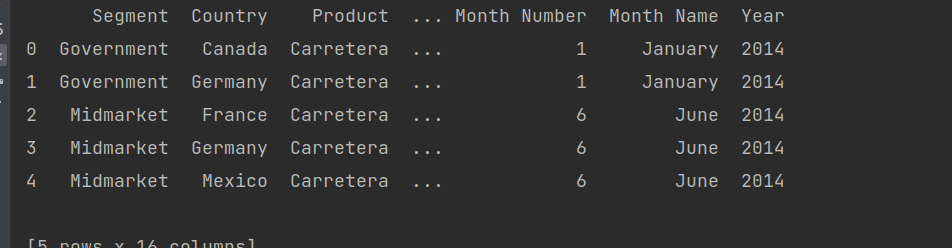If you want to print records from a particular index, we can use this method

`print(df[2:5])`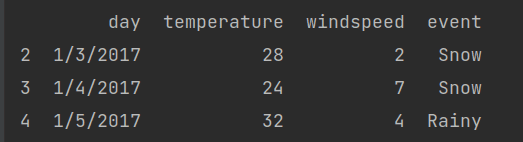The result holds the record of 2 to 4 index values

Like this, we can do operations on the columns too.

If you want to get the columns, then you need to use this code

`print(df.columns)`

Result:-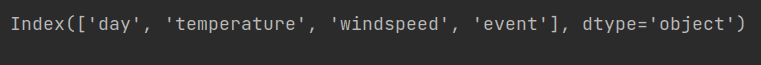If you need the individual column values, then you can print them by .column_name

`print(df.day)`

Here we get the day column values.If you want to print more than one column, we can achieve that by using this code.

`print(df[['day', 'event']])`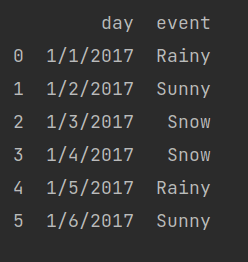Using the Pandas method, we can find the max, mean, average, etc

To find the maximum temperature, we can use this code

`print(df['temperature'].max())`

The result is.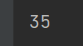Now let’s find the mean of the temperature

`print(df['temperature'].mean())`

Result: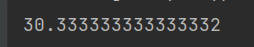Like this, there are many more function min(), std(), describe(), etc

This describe()  function helps to get the statistics like count, means, std, and so on.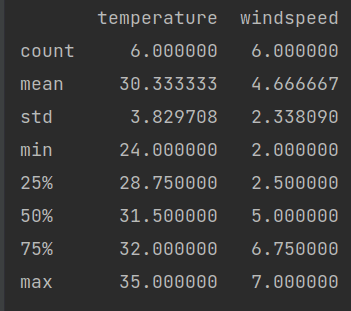For reading CSV files, we should give

`df = pd.read_csv(r'/home/cybrosys/Downloads/Financial Sample.csv)`

As we read the CSV files like that we have an option to_csv is used to store the CVS files in the system

`df.to_csv('demo.csv')`

Here, we have given the file name to be saved.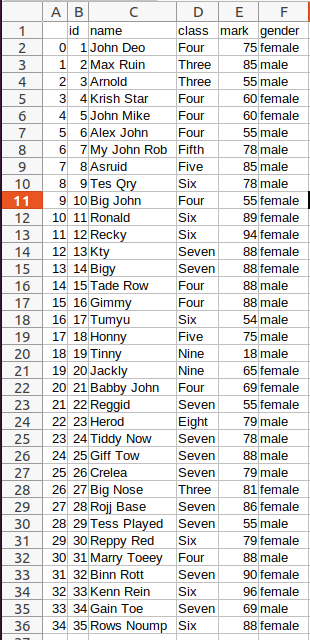If you want to remove the index while saving the file then you should use

`df.to_csv('demo.csv', index=False)`

These are some of the functions and methods for reading and writing an Excel or CVS file using pandas. Like this, there are many functions available in pandas. This blog will give you a basic understanding of how to read and write An Excel or CSV using Pandas library.

If you need any assistance in odoo, we are online, please chat with us.

### Recent Posts

#### How the Reception Report Works in Odoo 17 Inventory App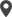Calicut

Cybrosys Technologies Pvt. Ltd.
Neospace, Kinfra Techno Park
Kakkancherry, Calicut
Kerala, India - 673635London

Cybrosys Limited
Alpha House,
100 Borough High Street, London,
SE1 1LB, United KingdomKochi

Cybrosys Technologies Pvt. Ltd.
1st Floor, Thapasya Building,
Kochi, India - 682030.Bangalore

Cybrosys Techno Solutions
The Estate, 8th Floor,